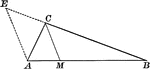### Angle Bisector of Triangle Dividing Opposite Side Into Proportionate Segments

Illustration to show that an angle bisector of a triangle divides the opposite side into segments which…### Exterior Angle Bisector of Triangle Dividing Opposite Side Into Proportionate Segments

Illustration to show that a bisector of a an exterior angle of a triangle divides the opposite side…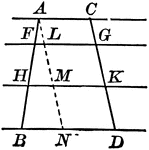### Parallel Lines Cut by Parallels, Corresponding Intercepts Proportional

Illustration to show that if parallel lines are cut by any number of parallels, the corresponding intercepts…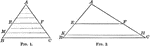### Triangle Divided by Parallel Lines to Form Proportionate Triangles

Illustration to show that if a line is drawn through two sides of a triangle parallel to the third side,…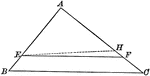### Triangle Divided by Parallel Lines to Form Proportionate Triangles

Illustration to show that if a line divides two sides of a triangle proportionally, it is parallel to…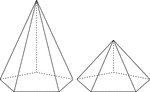### 2 Right Pentagonal Pyramids

Illustration of 2 right pentagonal pyramids with hidden edges shown. The pentagonal bases are congruent,…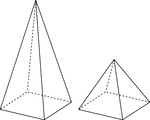### 2 Right Rectangular Pyramids

Illustration of 2 right rectangular pyramids with hidden edges shown. The rectangular bases are congruent,…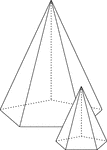### Similar Pentagonal Pyramids

Illustration of 2 similar right pentagonal pyramids with hidden edges shown. The height of the pyramid…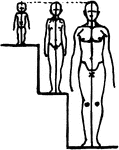### Relation of Head to Height by Age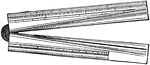### Sector

The sector is a hinged rule with scales used for various mathematical calculations.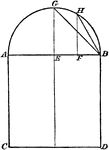### Construction of Proportional Square

Illustration used to construct a square that shall be in proportion to a given square.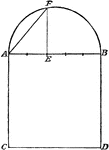### Construction of Proportional Square

Illustration used to construct a square that shall be in proportion to a given square.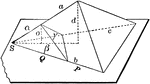### Two Proportional Tetrahedrons

Diagram used to prove the theorem: "Two tetrahedrons having a trihedral angle in each equal, are to…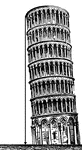### Leaning Tower

Illustration of a leaning tower with a perpendicular drawn from the top of the tower to the ground to…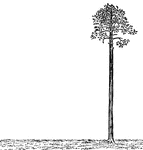### Palm Tree Perpendicular to Ground

Illustration of a palm tree that is perpendicular to the ground. The tree is perfectly straight, as…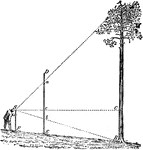### Using Proportions To Find Heights of Trees

Triangle diagram for measuring heights of trees using proportions.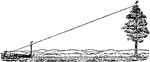### Using Proportions To Find Heights of Trees

Triangle diagram for measuring heights of trees using proportions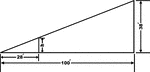### Inclined Plane Triangle

Inclined plane using right triangle and proportions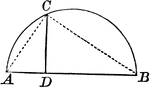### Right Triangle Inscribed in Semicircle Shows Mean Proportional

Right triangle inscribed in semicircle. Illustration shows that the perpendicular from any point in…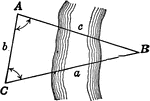### Oblique Triangle for Distance Across a River

Illustration of oblique triangle used to find distance across a river.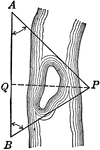### Oblique Triangle for Distance Across a River

Illustration of oblique triangle used to find distance across a river.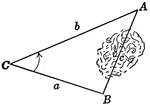### Oblique Triangle for Distance Across Lake

Illustration of oblique triangle used to find distance across a lake.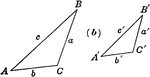### Similar Triangles

Similar triangles with corresponding sides labeled.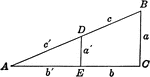### Similar Triangles

Similar triangles with corresponding sides labeled.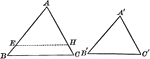### Mutually Equiangular Similar Triangles

Illustration that shows two mutually equiangular triangles are similar.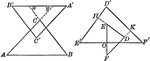### Similar Triangles With Respective Sides Parallel or Perpendicular

Illustration that shows that two triangles which have their sides respectively parallel, or respectively…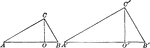### Similar triangles

Illustration of 2 similar triangles with altitudes drawn.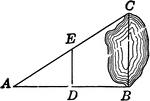### Similar Triangles for Distance Across Lake

Illustration of similar triangles used to find distance across a lake.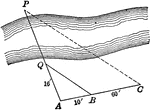### Similar Triangles for Distance Across Stream

Illustration of similar triangles used to find distance across a stream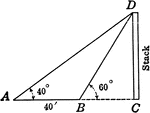### Similar Triangles for Height of Smokestack

Illustration of similar triangles used to find height of smokestack.### Similar Triangles With Altitudes Drawn

Illustration that shows two similar triangles with altitudes drawn.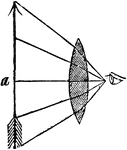### Visual Angle

"Suppose the object a, appears to the naked eye of the length repreesnted in the drawing. Now, as the…